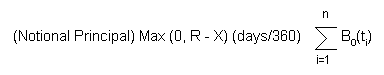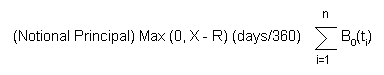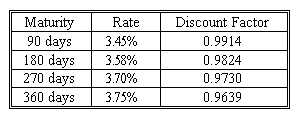### Why should I choose AnalystNotes?

Simply put: AnalystNotes offers the best value and the best product available to help you pass your exams.

### Subject 7. Interest rate swaption payoffs

At expiration, an interest rate payer swaption is worth the maximum of zero or the present value of the difference between the market swap rate and the exercise rate, valued as an annuity extending over the remaining life of the underlying swap. To value a receiver swaption at expiration, we take the difference between the exercise rate and the market swap rate, adjusted for its present value over the life of the underlying swap. These figures must be multiplied by the notional principal.

Example:

MPK considers the need to engage in a \$10 million three-year swap in two years. Worried about rising rates, it buys a payer swaption at an exercise rate of 11.5 percent. Swap payments will be annual.

At expiration, the following rates occur (Eurodollar zero coupon bond prices in parentheses):

• 360 day rate: 0.12 (0.8929).
• 720 day rate: 0.1328 (0.7901).
• 1080 day rate: 0.1451 (0.6967).

The rate on 3-year swaps is, therefore, R = [(1 - 0.6967) / (0.8929 + 0.7901 + 0.6967)] (360/360) = 0.1275.

So MPK could enter into a swap at 12.75 percent in the market or exercise the swaption and enter into a swap at 11.5 percent. Obviously it would exercise the swaption. What is the swaption worth?

Exercise would create a stream of 11.5 percent fixed payments and LIBOR floating receipts. MPK could then enter into the opposite swap in the market to receive 12.75 fixed and pay LIBOR floating. The LIBORs offset leaving a three-year annuity of 12.75 - 11.5 = 1.25 percent, or \$125,000 on \$10 million notional principal. The value of this stream of payments is \$125,000(0.8929 + 0.7901 + 0.6967) = \$297,463. Therefore a swaption effectively creates an annuity.

Alternatively, the holder can arrange to receive a new payment stream of \$125,000. In this case, the counterparty to the second swap is probably the same as the counterparty to the swap created by exercising the swaption, who would be the counterparty to the swaption. Because the floating payments are eliminated, the amount of cash passing between the parties is reduced, which mitigates the credit risk.

The holder can also receive an up-front cash payment of \$297,463.

Other than transaction costs and the credit risk associated with the newly created swaps, each of these means of exercising a swaption has the same value. Cash settlement is the most common approach at expiration.

Summary

The market value of a swaption at expiration can be received in one of four ways:

• By exercising the swaption to enter into the underlying swap.
• By exercising the swaption and entering into an offsetting swap that keeps both swaps in force.
• By exercising the swaption and entering into an offsetting swap that eliminates both swaps and pays a series of payments equal to the net difference in the fixed rates on the two swaps.
• By exercising the swaption and receiving a lump sum cash payment.

In general, the value of a payer swaption at expiration is:The value of a receiver swaption at expiration isThe Equivalence of Swaptions and Options on Bonds Using the above example, substituting the formula for the swap rate in the market, R, into the formula for the payoff of a swaption gives

Max [0, 1 - 0.6967 - 0.115(0.8929 + 0.7901 + 0.6967)]

This is the formula for the payoff of a put option on a bond with 11.5 percent coupon where the option has an exercise price of par. So payer swaptions are equivalent to puts on bonds.

Similarly, receiver swaptions are equivalent to calls on bonds.

Note that the mathematical formulas are presented in the textbook but not here as you do NOT need to remember them. It's always better to go through this with an example such as the above one.

#### Practice Question 1

A payer swaption is a ______ option on a bond and a receiver swaption is a ______ option on a bond.

A. call, put
B. put, call
C. put, put

#### Practice Question 2

Consider a European receiver swaption that expires in two years and is on a one-year swap that will make quarterly payments. The swaption has an exercise rate of 6.5%. The notional principal is \$100 million. At expiration, the term structure of interest rates is as follows:

L0(90) = 0.0373; L0(180) = 0.0429; L0(270) = 0.0477; L0(360) = 0.0538.

What is the market value of the swaption at expiration?

First we compute the present value discount factors:
B0(90) = 1 / (1 + 0.0373 (90/360)) = 0.9908.
B0(180) = 1 / (1 + 0.0429 (180/360)) = 0.9790.
B0(270) = 1 / (1 + 0.0477 (270/360)) = 0.9655.
B0(360) = 1 / (1 + 0.0538 (360/360)) = 0.9489.

The fixed rate should be: 1/(90/360) x (1 - 0.9489) / (0.9908 + 0.9790 + 0.9655 + 0.9489) = 0.0528.

The market value at expiration of the receiver swaption is Max {0, [0.065 x (90/360) - 0.0528 x (90/360)] x (0.9908 + 0.9790 + 0.9655 + 0.9489)} = 0.01184681.

Based on notional principal of \$100 million, the market value is \$100,000,000 x 0.01184681 = \$1,184,681.

#### Practice Question 3

Continue with question 2. Show that the payoff is equivalent to that of a call option on a bond with exercise price of \$1 (the par value of the bond).

Correct Answer: At expiration, the market value of a bond with face (exercise price) of \$1 and annual coupon of 6.5% is (0.065 x 90/360) x (0.9908 + 0.9790 + 0.9655 + 0.9489) + 1 (0.9489) = 1.012. The payoff on a call option on this bond with exercise price of \$1 is Max [0, (1.012 - 1)] = 0.012. This is the same as the payoff on the swaption.

#### Practice Question 4

Consider a European payer swaption that expires in two years and is exercisable into a one-year swap with quarterly payments, using 90/360 as the day-count adjustment. The exercise rate is 3.6%. The notional principal is \$20 million. Now suppose we are at the swaption expiration and the term structure is as follows:What is the value of the swaption right before the expiration?

A. \$15,643
B. \$4,000
C. \$184,000

Under the conditions, the swap fixed payment should be 0.0092, equating to an annual fixed rate of 3.68%. The holder of the swaption has the right to enter into a swap to pay 3.6% (which corresponds to a quarterly payment of \$180,000), whereas in the market such a swap would require payment at a rate of 3.68% (which corresponds to a quarterly payment of \$184,000). If the holder exercises the swap, and enters an opposite swap in the market, he effectively receives a net quarterly payment stream of \$184,000 - \$180,000 = \$4,000. The present value of this payment stream is \$4,000 (0.9914 + 0.9824 + 0.9730 + 0.9639) = \$15,643.

#### Practice Question 5

Consider a European payer swaption that expires in two years and is on a one-year swap that will make quarterly payments. The swaption has an exercise rate of 6.5%. The notional principal is \$100 million. At expiration, the term structure of interest rates is as follows:
L0(90) = 0.0373; L0(180) = 0.0429; L0(270) = 0.0477; L0(360) = 0.0538.

What is the market value of the swaption at expiration?

A. \$0.
B. \$1.2 million.
C. \$1.5 million.